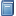Buchempfehlung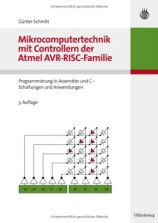Mikrocomputertechnik mit Controllern der Atmel AVR-RISC-Familie
Umfassend, aber leicht verständlich führt dieses Buch in die Programmierung von ATMEL AVR Mikrocontrollern ein. [Mehr Infos...]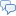FreeBASIC-Chat
Es sind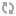Benutzer im FreeBASIC-Chat online.
(Stand:  )

# fb:porticula NoPaste

## CheckBox.bi

 Uploader: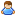OneCypher Datum/Zeit: 13.10.2009 10:58:27
Hinweis: Dieser Quelltext ist Bestandteil des Projekts GuiPtr, zu dem es auf FreeBASIC-Portal.de eine Projektseite gibt.

```#include once "GuiPtr.bi" Type CheckBox     Object as GuiObject ptr     Value as integer     Caption as String     declare Constructor(left as integer, top as integer, CBCaption as string, CBValue as integer = 1<>1) end type Sub DrawCheckBox(GO as any ptr)     dim CB as CheckBox ptr = GO     with *CB->Object         draw string .Buffer,(.left+ 17, .top+1), CB->Caption, RGB(0,0,0)         line .Buffer, (.left,.top)-(.left+14,.top + 14),RGB(0,0,0),B         if CB->Value then             line .Buffer, (.left+2,.top+2)-(.left+12,.top + 12),RGB(0,0,0)             line .Buffer, (.left+12,.top+2)-(.left+2,.top + 12),RGB(0,0,0)         end if     end with end sub Sub CBChangeValue(GO as any ptr, e as EventParameter)     dim CB as CheckBox ptr = GO     if CB->Value then         CB->Value = 1 <> 1     else         CB->Value = 1 <> 0     end if end sub Constructor CheckBox(left as integer, top as integer, CBCaption as string, CBValue as integer = 1<>1)     if CBValue = 1 then CBValue = 1<>0     Object = New GuiObject(@This)     with *Object         .ClassName = "CheckBox"         .left = left         .top = top         .width = 16 + (len(CBCaption) * 8)         .height = 14         .PrivateEvents = new Events         with *.PrivateEvents             .OnDraw = @DrawCheckBox             .SingleClick = @CBChangeValue             .DoubleClick = @CBChangeValue         end with     end with     Caption = CBCaption     Value = CBValue end constructor```